Starts With A Bang

# This Is How Physics, Not Math, Finally Resolves Zeno’s Famous Paradox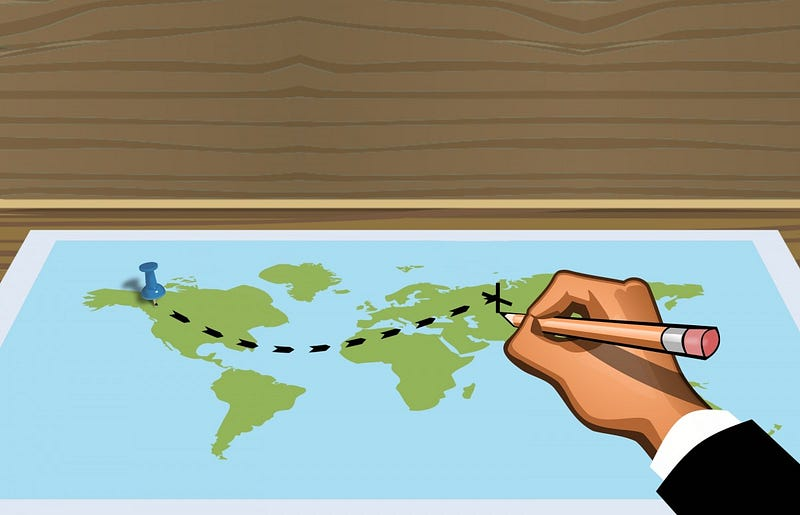If you want to travel a finite distance, you first have to travel half that distance. If you keep halving the distance, you’ll require an infinite number of steps. Does that mean motion is impossible? (PXHERE / PUBLIC DOMAIN)

#### Zeno’s paradox stumped philosophers, mathematicians, and intellectuals for millennia. It took physics to finally solve it.

The fastest human in the world, according to the Ancient Greek legend, was the heroine Atalanta. Although she was a famous huntress who even joined Jason and the Argonauts in the search for the golden fleece, she was renowned for her speed, as no one could defeat her in a fair footrace. But she was also the inspiration for the first of many similar paradoxes put forth by the ancient philosopher Zeno of Elea: about how motion, logically, should be impossible.

To go from her starting point to her destination, Atalanta must first travel half of the total distance. To travel the remaining distance, she must first travel half of what’s left over. No matter how small a distance is still left, she must travel half of it, and then half of what’s still remaining, and so on, ad infinitum. With an infinite number of steps required to get there, clearly she can never complete the journey. And hence, Zeno states, motion is impossible: Zeno’s paradox. Here’s the unintuitive resolution.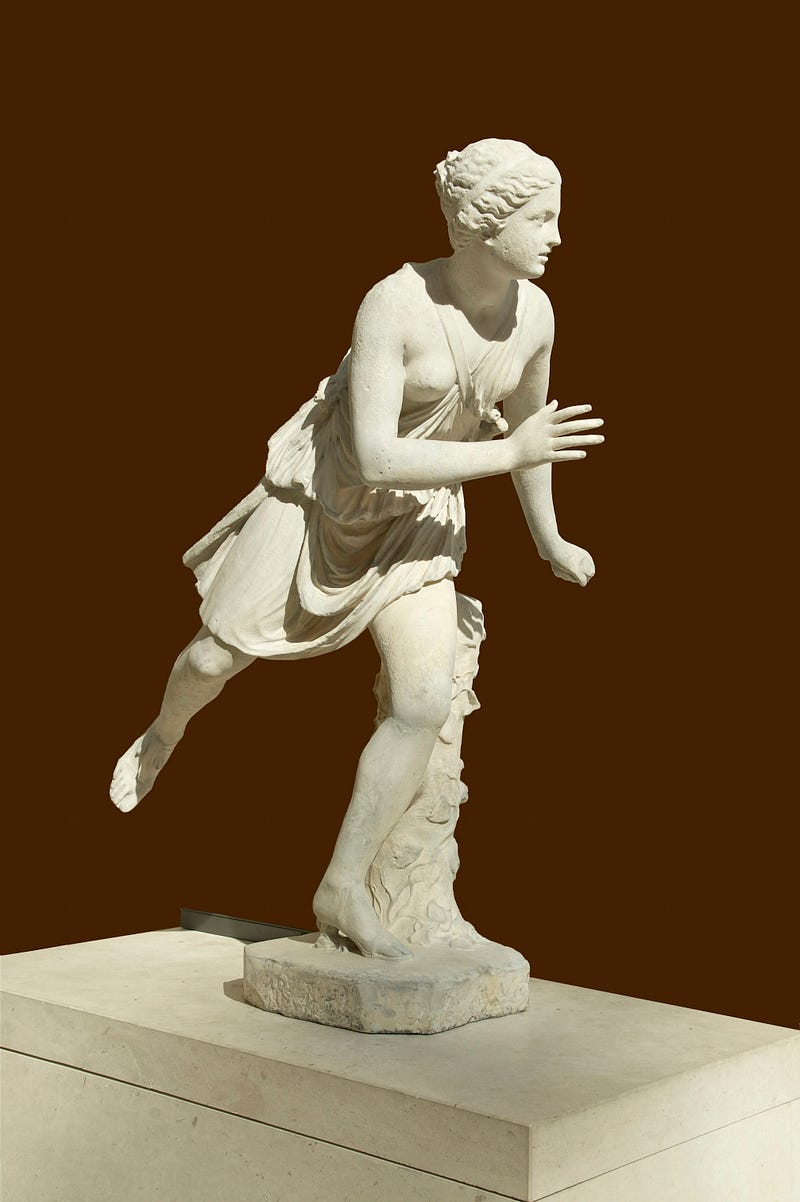A scuplture of Atalanta, the fastest person in the world, running in a race. If not for the trickery of Aphrodite and the allure of the three golden apples, nobody could have defeated Atalanta in a fair footrace. (JEBULON / WIKIMEDIA COMMONS)

The oldest “solution” to the paradox was done from a purely mathematical perspective. The claim admits that, sure, there might be an infinite number of jumps that you’d need to take, but that each new jump got smaller and smaller than the prior one. Therefore, as long as you could demonstrate that the total sum of every jump you need to take adds up to a finite value, it doesn’t matter how many chunks you divide it into.

For example, if the total journey is defined to be 1 unit (whatever that unit is), then you could get there by adding half after half after half, etc. The series ½ + ¼ + ⅛ + … does indeed converge to 1, so that you wind up covering the entire needed distance if you add an infinite number of terms. You can prove this, cleverly, by subtracting the entire series from double the entire series as follows:

• (series) = ½ + ¼ + ⅛ + …
• 2 * (series) = 1 + ½ + ¼ + ⅛ + …
• Therefore, [2 * (series) — (series)] = 1 + (½ + ¼ + ⅛ + …) — (½ + ¼ + ⅛ + …) = 1.

Simple, straightforward, and compelling, right?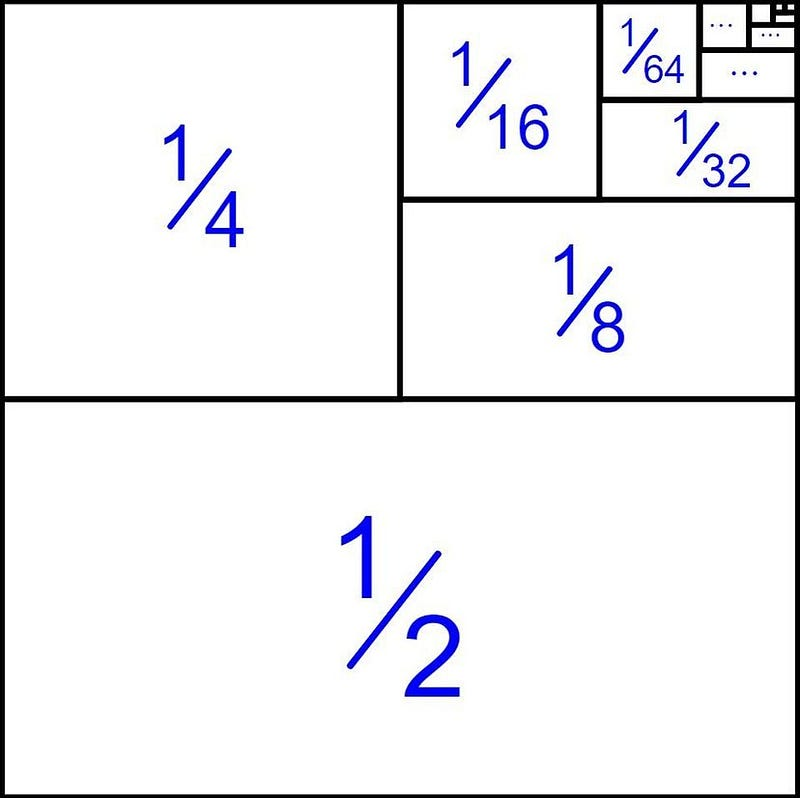By continuously halving a quantity, you can show that the sum of each successive half leads to a convergent series: one entire “thing” can be obtained by summing up one half plus one fourth plus one eighth, etc. (PUBLIC DOMAIN IMAGE)

But it’s also flawed. This mathematical line of reasoning is only good enough to show that the total distance you must travel converges to a finite value. It doesn’t tell you anything about how long it takes you to reach your destination, and that’s the tricky part of the paradox.Travel the Universe with astrophysicist Ethan Siegel. Subscribers will get the newsletter every Saturday. All aboard!

How could time come into play to ruin this mathematically elegant and compelling “solution” to Zeno’s paradox?

Because there’s no guarantee that each of the infinite number of jumps you need to take — even to cover a finite distance — occurs in a finite amount of time. If each jump took the same amount of time, for example, regardless of the distance traveled, it would take an infinite amount of time to cover whatever tiny fraction-of-the-journey remains. Under this line of thinking, it may still be impossible for Atalanta to reach her destination.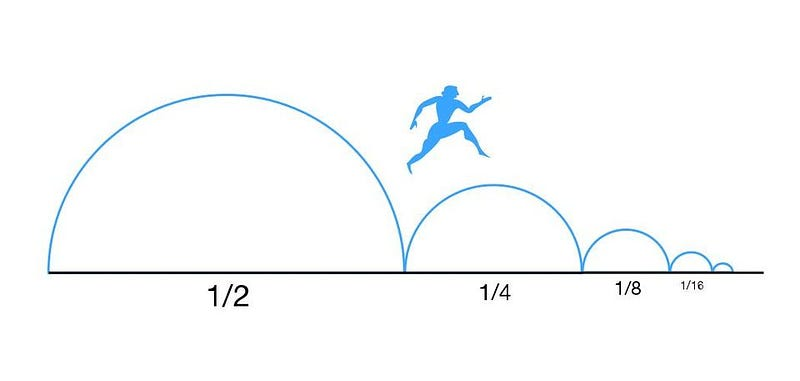One of the many representations (and formulations) of Zeno of Elea’s paradox relating to the impossibility of motion. It was only through a physical understanding of distance, time, and their relationship that this paradox was resolved. (MARTIN GRANDJEAN / WIKIMEDIA COMMONS)

Many thinkers, both ancient and contemporary, tried to resolve this paradox by invoking the idea of time. Specifically, as asserted by Archimedes, it must take less time to complete a smaller distance jump than it does to complete a larger distance jump, and therefore if you travel a finite distance, it must take you only a finite amount of time. And therefore, if that’s true, Atalanta can finally reach her destination and complete her journey.

Only, this line of thinking is flawed, too. It’s eminently possible that the time it takes to finish each step will still go down: half the original time, a third of the original time, a quarter of the original time, a fifth, etc., but that the total journey will take an infinite amount of time. You can check this for yourself by trying to find what the series [½ + ⅓ + ¼ + ⅕ + ⅙ + …] sums to. As it turns out, the limit does not exist: this is a diverging series.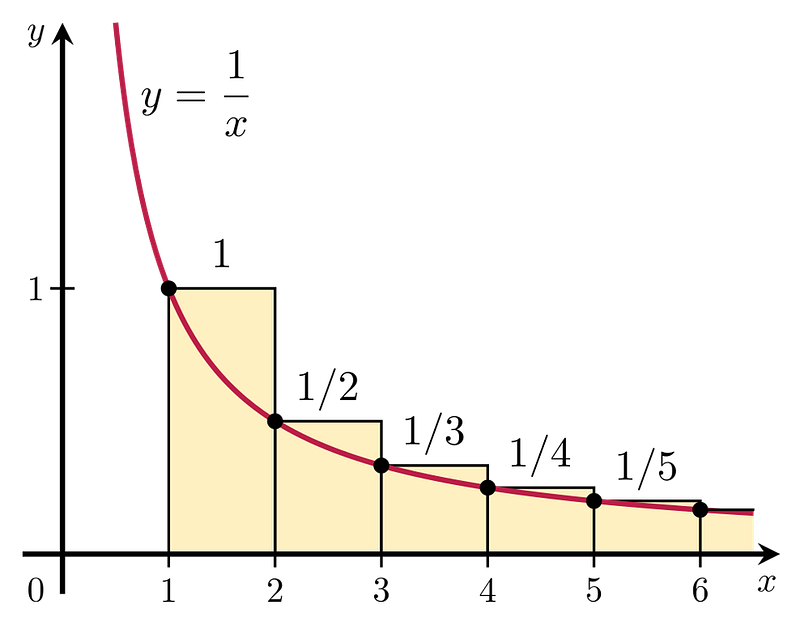The harmonic series, as shown here, is a classic example of a series where each and every term is smaller than the previous term, but the total series still diverges: i.e., has a sum that tends towards infinity. It is not enough to contend that time jumps get shorter as distance jumps get shorter; a quantitative relationship is necessary. (PUBLIC DOMAIN)

It might seem counterintuitive, but pure mathematics alone cannot provide a satisfactory solution to the paradox. The reason is simple: the paradox isn’t simply about dividing a finite thing up into an infinite number of parts, but rather about the inherently physical concept of a rate.

Although the paradox is usually posed in terms of distances alone, the paradox is really about motion, which is about the amount of distance covered in a specific amount of time. The Greeks had a word for this concept — τάχος — which is where we get modern words like “tachometer” or even “tachyon” from, and it literally means the swiftness of something. But this concept was only known in a qualitative sense: the explicit relationship between distance and “τάχος,” or velocity, required a physical connection: through time.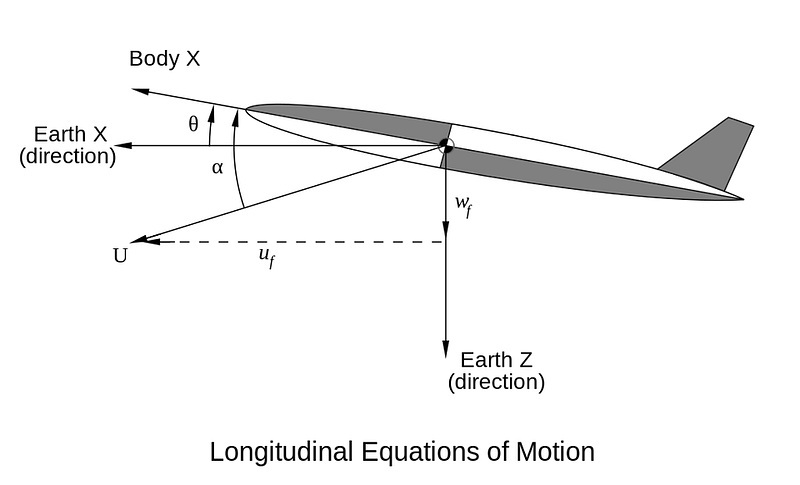If anything moves at a constant velocity and you can figure out its velocity vector (magnitude and direction of its motion), you can easily come up with a relationship between distance and time: you will traverse a specific distance in a specific and finite amount of time, depending on what your velocity is. This can be calculated even for non-constant velocities by understanding and incorporating accelerations, as well, as determined by Newton. (GORDON VIGURS / ENGLISH WIKIPEDIA)

How fast does something move? That’s a speed.

Add in which direction it’s moving in, and that becomes velocity.

And what’s the quantitative definition of velocity, as it relates to distance and time? It’s the overall change in distance divided by the overall change in time.

This is a concept known as a rate: the amount that one quantity (distance) changes as another quantity (time) changes as well. You can have a constant velocity (without acceleration) or a changing velocity (with acceleration). You can have an instantaneous velocity (your velocity at one specific moment in time) or an average velocity (your velocity over a certain part or whole of a journey).

But if something is in constant motion, the relationship between distance, velocity, and time becomes very simple: distance = velocity * time.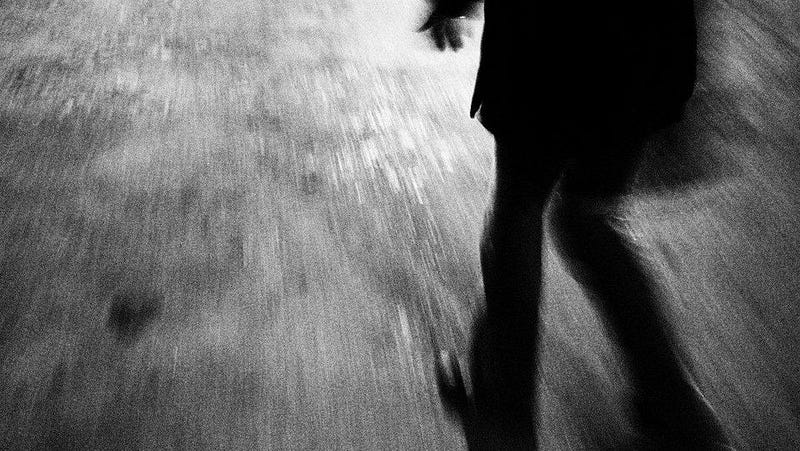When a person moves from one location to another, they are traveling a total amount of distance in a total amount of time. Figuring out the relationship between distance and time quantitatively did not happen until the time of Galileo and Newton, at which point Zeno’s famous paradox was resolved not by mathematics or logic or philosophy, but by a physical understanding of the Universe. (PUBLIC DOMAIN)

This is the resolution of the classical “Zeno’s paradox” as commonly stated: the reason objects can move from one location to another (i.e., travel a finite distance) in a finite amount of time is because their velocities are not only always finite, but because they do not change in time unless acted upon by an outside force. If you take a person like Atalanta moving at a constant speed, she will cover any distance in an amount of time put forth by the equation that relates distance to velocity.

This is basically Newton’s first law (objects at rest remain at rest and objects in motion remain in constant motion unless acted on by an outside force), but applied to the special case of constant motion. If you halve the distance you’re traveling, it takes you only half the time to traverse it. To travel (½ + ¼ + ⅛ + …) the total distance you’re trying to cover, it takes you (½ + ¼ + ⅛ + …) the total amount of time to do so. And this works for any distance, no matter how arbitrarily tiny, you seek to cover.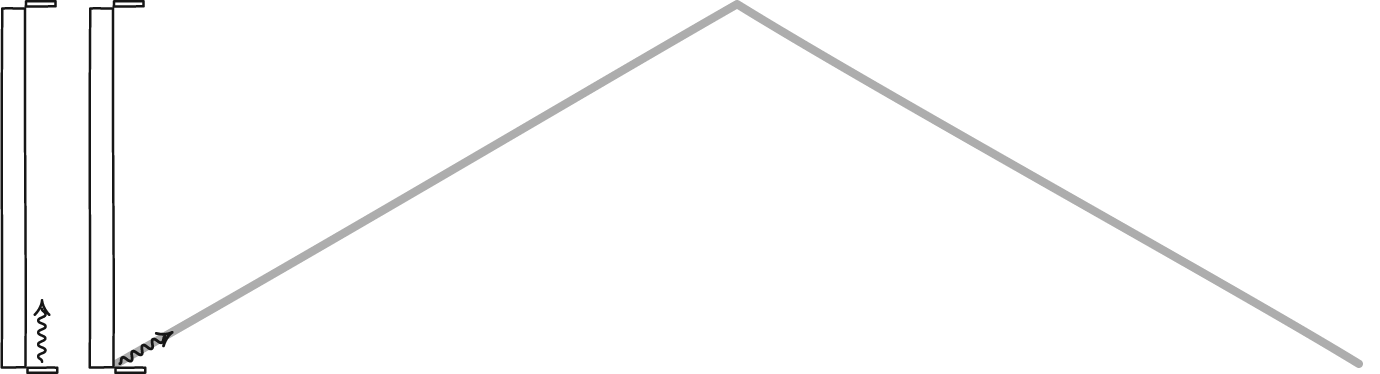Whether it’s a massive particle or a massless quantum of energy (like light) that’s moving, there’s a straightforward relationship between distance, velocity, and time. If you know how fast your object is going, and if it’s in constant motion, distance and time are directly proportional. (JOHN D. NORTON, VIA HTTP://WWW.PITT.EDU/~JDNORTON/TEACHING/HPS_0410/CHAPTERS/SPECIAL_RELATIVITY_CLOCKS_RODS/)

For anyone interested in the physical world, this should be enough to resolve Zeno’s paradox. It works whether space (and time) is continuous or discrete; it works at both a classical level and a quantum level; it doesn’t rely on philosophical or logical assumptions. For objects that move in this Universe, physics solves Zeno’s paradox.

But at the quantum level, an entirely new paradox emerges, known as the quantum Zeno effect. Certain physical phenomena only happen due to the quantum properties of matter and energy, like quantum tunneling through a barrier or radioactive decays. In order to go from one quantum state to another, your quantum system needs to act like a wave: its wavefunction spreads out over time.

Eventually, there will be a non-zero probability of winding up in a lower-energy quantum state. This is how you can tunnel into a more energetically favorable state even when there isn’t a classical path that allows you to get there.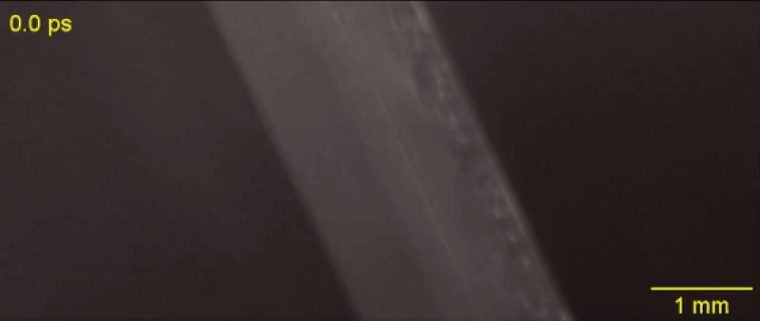By firing a pulse of light at a semi-transparent/semi-reflective thin medium, researchers can measure the time it must take for these photons to tunnel through the barrier to the other side. Although the step of tunneling itself may be instantaneous, the traveling particles are still limited by the speed of light. (J. LIANG, L. ZHU & L. V. WANG, LIGHT: SCIENCE & APPLICATIONSVOLUME 7, 42 (2018))

But there’s a way to inhibit this: by observing/measuring the system before the wavefunction can sufficiently spread out. Most physicists refer to this type of interaction as “collapsing the wavefunction,” as you’re basically causing whatever quantum system you’re measuring to act “particle-like” instead of “wave-like.” But that’s just one interpretation of what’s happening, and this is a real phenomenon that occurs irrespective of your chosen interpretation of quantum physics.

What’s actually occurring is that you’re restricting the possible quantum states your system can be in through the act of observation and/or measurement. If you make this measurement too close in time to your prior measurement, there will only be an infinitesimal (or even a zero) probability of tunneling into your desired state. If you keep your quantum system interacting with the environment, you can suppress the inherently quantum effects, leaving you with only the classical outcomes as possibilities.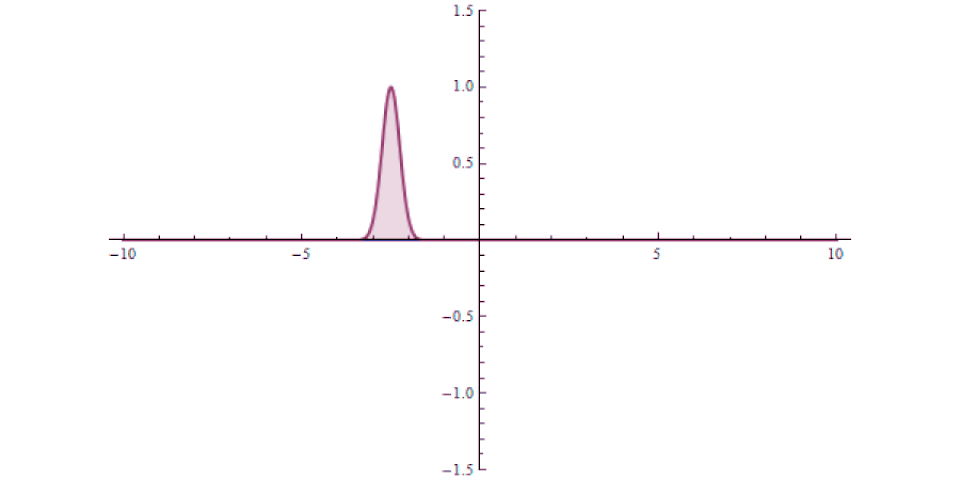When a quantum particle approaches a barrier, it will most frequently interact with it. But there is a finite probability of not only reflecting off of the barrier, but tunneling through it. If you were to measure the position of the particle continuously, however, including upon its interaction with the barrier, this tunneling effect could be entirely suppressed via the quantum Zeno effect. (YUVALR / WIKIMEDIA COMMONS)

The takeaway is this: motion from one place to another is possible, and it’s because of the explicit physical relationship between distance, velocity and time that we can learn exactly how motion occurs in a quantitative sense. Yes, in order to cover the full distance from one location to another, you have to first cover half that distance, then half the remaining distance, then half of what’s left, etc.

But the time it takes to do so also halves, and so motion over a finite distance always takes only a finite amount of time for any object in motion. Although this is still an interesting exercise for mathematicians and philosophers, not only is the solution reliant on physics, but physicists have even extended it to quantum phenomena, where a new quantum Zeno effect — not a paradox, but a suppression of purely quantum effects — emerges. As in all scientific fields, the Universe itself is the final arbiter of how reality behaves. Thanks to physics, we at last understand how.

Ethan Siegel is the author of Beyond the Galaxy and Treknology. You can pre-order his third book, currently in development: the Encyclopaedia Cosmologica.

Related

# 7 great but notoriously hard-to-finish books

These hard-to-finish books are still worth the effort.

# Pando, the world’s largest organism, has stopped growing

Pando is a stand of aspen in Utah that is 14,000 years old and weighs 12 million pounds. Humans threaten to end its long reign.

# 1,000-year-old stalagmites in Indian cave reveal a history of long, deadly droughts

The monsoon rains were not always so reliable.

# The credibility of science is damaged when universities brag about themselves

The “attention economy” corrupts science.

# Was the mysterious, \$450-million “Salvator Mundi” really painted by Leonardo da Vinci?

“Salvator Mundi” sold for a record-breaking \$450 million in 2017, but is it really as valuable as people were led to believe?

Up Next

# For a child, being carefree is intrinsic to a well-lived life

Do adults need to be more carefree, in order for their lives to go well?